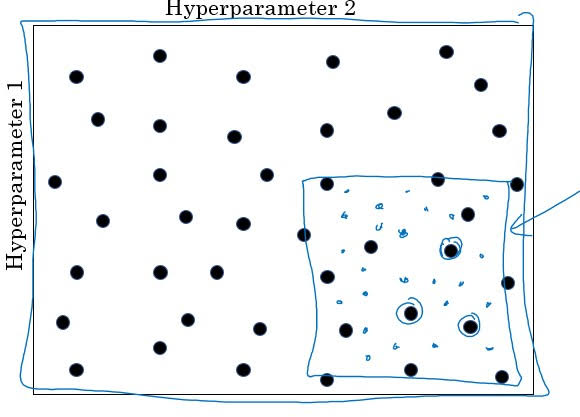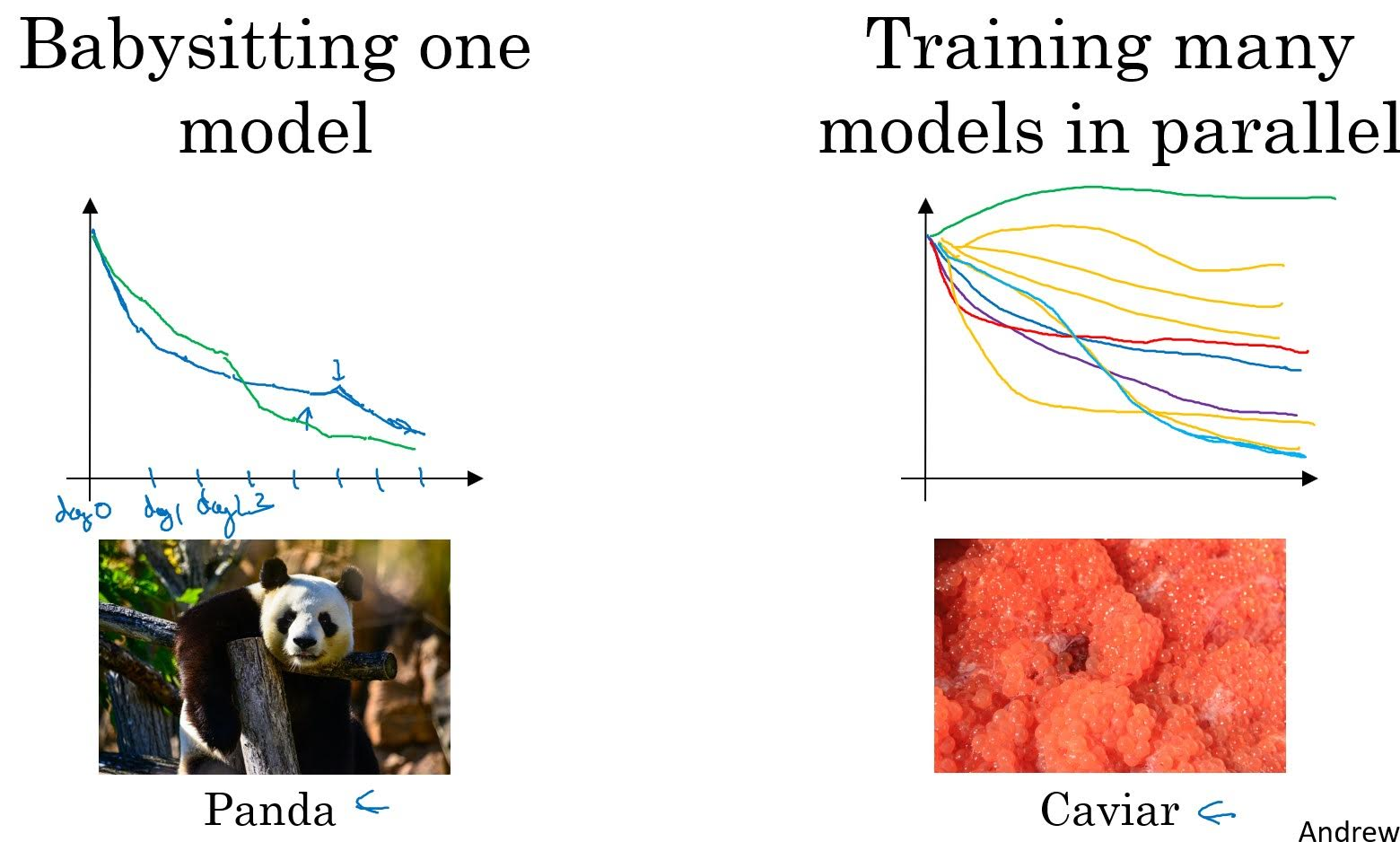# Hyperparameter Tuning

Categories:

Updated:

Parameters like $W$ and $b$ were trainable with gradient descent. However we should tune hyperparmeters like #units, #layers, learning rate and so on with evaluation on validatation set.

## Try random values; don’t use a grid

For example, when picking number of layers, you can randomly pick 6 numbers from range 1 to 50 for validating, rather than testing every 50 numbers.

After testing like above, assume you’ve found out 10 layers and 19 layers did well. Then you can randomly pick 3 numbers from range 10 to 19 for validation.## Use appropriate scale to pick hyperparmeters

When randomly picking $n$ values from a range of values(from a to b), you need to set appropriate scale.

1. Linear Scale
• When picking #units or #layers: $np.random.randint(a, b, n)$
2. Log Scale
• When picking learning rate: $r = np.random.rand(n)*(b-a)+a$
$\alpha = 10^r$
• When picking $\beta$ for momentum or RMSProp: $r=np.random.rand(n)*(b-a)+a$
$\beta=1-10^r$

## Tune occasionally

Environments can change after starting servicing with a model. You have to re-evaluate your model and re-tune hyperparmeters occasionally to adapt to new environments.

## Use learning curve to pick hyperparameters

If you have enough computing power, you can train many models of different hyperparmeters and choose one with best performance based on their learning curves.

If you don’t have enough computing power, you can train one model with a set of hyperparmeteres, and see it’s learning curve while training. Based on your observasion, you can ocasionally stop training and change hyperparemeters and resume training. However, in this case you can’t change architecture of a neural network such as number of layers or units.Categories: